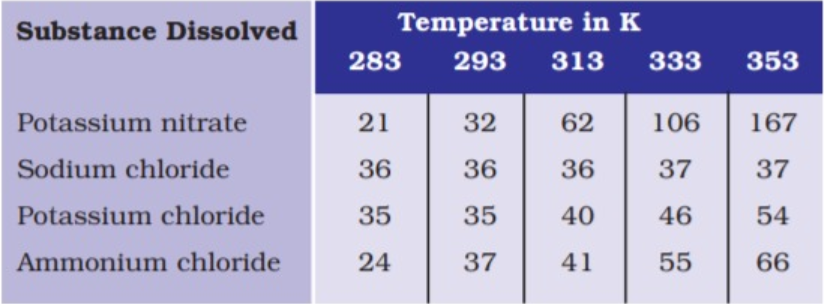# Pragya tested the solubility of three different substances at different temperatures and collected the data as given below (results are given in the f

58 views

closed
Pragya tested the solubility of three different substances at different temperatures and collected the data as given below (results are given in the following table, as grams of substance dissolved in 100 grams of water to form a saturated solution).(a) What mass of potassium nitrate would be needed to produce a saturated solution of potassium nitrate in 50 grams of water at 313 K ?
(b) Pragya makes a saturated solution of potassium chloride in water at 353 K and leaves the solution to cool at room temperature. What would she observe as the solution cools ? Explain.
(c ) Find the solubility of each salt at 293 K . Which salt has the highest solubility at this temperature ?
(d) What is the effect of change of temperature on the solubility of a salt ?

by (49.8k points)
selected by

(a) Since 62 g of potassium nitrate is dissolved in 100 g of water to prepare a saturated at 313 K, 31 g of potassium nitrate should be dissolved in 50 g of water to prepare a saturate solution at 313 K.
(b) The amount of potassium chloride that should be dissolved in water to make a saturated solution increases with temperature. Thus, as the solution cools some of the potassium chloride will precipitate out of the solution.
(c ) The solubility of the salts at 293 K are :
Potassium nitrate-32 g
Sodium chloride - 36 g
Potassium chloride - 35 g
Ammonium chloride - 37 g
Ammonium chloride has the highest solubility at 293 K.
(d ) the solubility of a salt increases with temperature.